Karel's DNN implementation

This documentation covers Karel Vesely's version of deep neural network code in Kaldi.

For an overview of all deep neural network code in Kaldi, see Deep Neural Networks in Kaldi, and for Dan's version, see Dan's DNN implementation.

The goal of this documentation is to provide useful information about the DNN recipe, and briefly describe neural network training tools. We'll start from the Top-level script, explain what happens in the Training script internals, show some Advanced features, and do a light introduction to the The C++ code with the focus on explaining how to extend it.

# Top-level script

Let's have a look at the script egs/wsj/s5/local/nnet/run_dnn.sh. This script assumes to use a single CUDA GPU, and that kaldi was compiled with CUDA (check for 'CUDA = true' in src/kaldi.mk). Also we assume that 'cuda_cmd' is set properly in egs/wsj/s5/cmd.sh either to a GPU cluster node using 'queue.pl' or to a local machine using 'run.pl'. And finally the script assumes we already have a SAT GMM system exp/tri4b and corresponding fMLLR transforms, as generated by egs/wsj/s5/run.sh. Note that for other databases the run_dnn.sh is typically in the same location s5/local/nnet/run_dnn.sh.

The script egs/wsj/s5/local/nnet/run_dnn.sh is split into several stages:

0. storing 40-dimensional fMLLR features to disk, steps/nnet/make_fmllr_feats.sh, this simplifies the training scripts, the 40-dimensional features are MFCC-LDA-MLLT-fMLLR with CMN

1. RBM pre-training, steps/nnet/pretrain_dbn.sh, implemented according to Geoff Hinton's tutorial paper. The training algorithm is Contrastive Divergence with 1-step of Markov Chain Monte Carlo sampling (CD-1). The first RBM has Gaussian-Bernoulli units, and following RBMs have Bernoulli-Bernoulli units. The hyper-parameters of the recipe were tuned on the 100h Switchboard subset. If smaller databases are used, mainly the number of epochs N needs to be set to 100h/set_size. The training is unsupervised, so it is sufficient to provide single data-directory with input features.

When training the RBM with Gaussian-Bernoulli units, there is a high risk of weight-explosion, especially with larger learning rates and thousands of hidden neurons. To avoid weight-explosion we implemented a mechanism, which compares the variance of training data with the variance of the reconstruction data in a minibatch. If the variance of reconstruction is >2x larger, the weights are shrinked and the learning rate is temporarily reduced.

2. Frame cross-entropy training, steps/nnet/train.sh, this phase trains a DNN which classifies frames into triphone-states (i.e. PDFs). This is done by mini-batch Stochastic Gradient Descent. The default is to use Sigmoid hidden units, Softmax output units and fully connected layers AffineTransform. The learning rate is 0.008, size of minibatch 256; we use no momentum or regularization (note.: the optimal learning-rate differs with type of hidden units, the value for sigmoid is 0.008, for tanh 0.00001)

The input_transform and the pre-trained DBN (i.e. Deep Belief Network, stack of RBMs) is passed into to the script using the options '–input-transform' and '–dbn', only the output layer is initialized randomly. We use early stopping to prevent over-fitting, for this we measure the objective function on the cross-validation set (i.e. held-out set), therefore two pairs of feature-alignment dirs are needed to perform the supervised training.

A good summary paper on DNN training is http://research.google.com/pubs/archive/38131.pdf

3.,4.,5.,6. sMBR sequence-discriminative training, steps/nnet/train_mpe.sh, this phase trains the neural network to jointly optimize for whole sentences, which is closer to the general ASR objective than frame-level training.

• The objective of sMBR is to maximize the expected accuracy of state labels derived from the reference transcription alignment, while a lattice framework is used to represent competing hypothesis.
• The training is done by Stochastic Gradient Descent with per-utterance updates, we use a low learning rate 1e-5 (sigmoids) which is kept constant and we run 3-5 epochs.
• We have observed faster convergence when re-generating lattices after the 1st epoch. We support MMI, BMMI, MPE and sMBR training. All the techniques performed very similar on the Switchboard 100h set, only sMBR was a little better.
• In sMBR optimization we exclude silence frames from accumulating approximate accuracies. A more detailed description is at http://www.danielpovey.com/files/2013_interspeech_dnn.pdf

Other interesting top-level scripts:

Besides the DNN recipe, there are also other example scripts which can be handy:

• DNN : egs/wsj/s5/local/nnet/run_dnn.sh , (main top-level script)
• CNN : egs/rm/s5/local/nnet/run_cnn.sh , (CNN = Convolutional Neural Network, see paper, we have 1D convolution on frequency axis)
• Autoencoder training : egs/timit/s5/local/nnet/run_autoencoder.sh
• Tandem system : egs/swbd/s5c/local/nnet/run_dnn_tandem_uc.sh , (uc = Universal context network, see paper)
• Multilingual/Multitask : egs/rm/s5/local/nnet/run_blocksoftmax.sh, (Network with <BlockSoftmax> output trained on RM and WSJ, same C++ design as was used in SLT2012 paper)

# Training script internals

The main neural network training script steps/nnet/train.sh is invoked as:

steps/nnet/train.sh <data-train> <data-dev> <lang-dir> <ali-train> <ali-dev> <exp-dir>


The network input features are taken from data directories <data-train> <data-dev>, the training targets are taken from directories <ali-train> <ali-dev>. The <lang-dir> is used only in the special case when using LDA feature-transform, and to generate phoneme frame-count statistics from the alignment, it is not crucial for the training. The output (i.e. the trained networks and logfiles) goes into <exp-dir>.

Internally the script prepares the feature+target pipelines, generates a neural-network prototype and initialization, creates feature_transform and calls the scheduler script steps/nnet/train_scheduler.sh, which runs the training epochs and controls the learning rate.

While looking inside steps/nnet/train.sh we see:

1. CUDA is required, the scripts exit if no GPU was detected or was CUDA not compiled in (one can still use '–skip-cuda-check true' to run on CPU, but it is 10-20x slower)

2. alignment pipelines get prepared, the training tool requires targets in Posterior format, hence ali-to-post.cc is used:

labels_tr="ark:ali-to-pdf $alidir/final.mdl \"ark:gunzip -c$alidir/ali.*.gz |\" ark:- | ali-to-post ark:- ark:- |"
labels_cv="ark:ali-to-pdf $alidir/final.mdl \"ark:gunzip -c$alidir_cv/ali.*.gz |\" ark:- | ali-to-post ark:- ark:- |"


3. shuffled features get copied to /tmp/???/..., this can be disabled by '–copy-feats false', or location changed by '–copy-feats-tmproot <dir>'

• The features are re-saved to disk using the shuffled list, this dramatically lowers the stress on hard-disks during training, as it prevents from heavy disk-seeking

4. the feature pipeline is prepared:

# begins with copy-feats:
feats_tr="ark:copy-feats scp:$dir/train.scp ark:- |" feats_cv="ark:copy-feats scp:$dir/cv.scp ark:- |"
# optionally apply-cmvn is appended:
feats_tr="$feats_tr apply-cmvn --print-args=false --norm-vars=$norm_vars --utt2spk=ark:$data/utt2spk scp:$data/cmvn.scp ark:- ark:- |"
feats_cv="$feats_cv apply-cmvn --print-args=false --norm-vars=$norm_vars --utt2spk=ark:$data_cv/utt2spk scp:$data_cv/cmvn.scp ark:- ark:- |"
feats_tr="$feats_tr add-deltas --delta-order=$delta_order ark:- ark:- |"
feats_cv="$feats_cv add-deltas --delta-order=$delta_order ark:- ark:- |"


5. feature_transform is prepared :

• feature_transform is a fixed function performed on the DNN front-end, it is computed by GPU. Usually it performs a type of dimensionality expansion. This allows to have low-dimensional features on hard-disk and high-dimensional features on DNN front-end. Saving both hard-disk space and read throughput.
• most of the nnet-binaries have the option '–feature-transform',
• what is generated depends on the option '–feat-type', the values are (plain|traps|transf|lda)

6. a network prototype is generated in utils/nnet/make_nnet_proto.py:

• each component is on a separate line, where dimensions and initialization hyper-parameters are specified
• for AffineTransform the bias is initialized by Uniform distribution given by <BiasMean> and <BiasRange>, while weights are initialized from Normal distribution scaled by <ParamStddev>
• note.: if you like to experiment with externally prepared prototypes, use option '–mlp-proto <proto>'
$cat exp/dnn5b_pretrain-dbn_dnn/nnet.proto <NnetProto> <AffineTransform> <InputDim> 2048 <OutputDim> 3370 <BiasMean> 0.000000 <BiasRange> 0.000000 <ParamStddev> 0.067246 <Softmax> <InputDim> 3370 <OutputDim> 3370 </NnetProto>  7. the network is initialized by : nnet-initialize.cc , the DBN gets prepended in the next step using nnet-concat.cc 8. finally the training gets called by running scheduler script steps/nnet/train_scheduler.sh Note : both neural networks and feature transforms can be viewed by nnet-info.cc, or shown in ascii by nnet-copy.cc While looking inside steps/nnet/train_scheduler.sh we see: the initial cross-validation run and the main for-loop over$iter which runs the epochs and controls the learning rate. Typically, the train_scheduler.sh is called from train.sh.

• the default learning-rate scheduling is based on the relative improvement of the objective function:
• initially the learning rate is kept constant if the improvement is larger than 'start_halving_impr=0.01',
• then the learning rate is reduced by multiplying with 'halving_factor=0.5' on each epoch,
• finally, the training is terminated if the improvement is smaller than 'end_halving_impr=0.001'

The neural networks get stored into $dir/nnet, logs are stored in$dir/log:

1. The network names contain record of epoch number (iter), learning-rate, and objective function value on training and cross-validation set (i.e. held-out set)

• We see that learning-rate halving started at 5th iter (i.e. epoch), which is a common case
$ls exp/dnn5b_pretrain-dbn_dnn/nnet nnet_6.dbn_dnn_iter01_learnrate0.008_tr1.1919_cv1.5895 nnet_6.dbn_dnn_iter02_learnrate0.008_tr0.9566_cv1.5289 nnet_6.dbn_dnn_iter03_learnrate0.008_tr0.8819_cv1.4983 nnet_6.dbn_dnn_iter04_learnrate0.008_tr0.8347_cv1.5097_rejected nnet_6.dbn_dnn_iter05_learnrate0.004_tr0.8255_cv1.3760 nnet_6.dbn_dnn_iter06_learnrate0.002_tr0.7920_cv1.2981 nnet_6.dbn_dnn_iter07_learnrate0.001_tr0.7803_cv1.2412 ... nnet_6.dbn_dnn_iter19_learnrate2.44141e-07_tr0.7770_cv1.1448 nnet_6.dbn_dnn_iter20_learnrate1.2207e-07_tr0.7769_cv1.1446 nnet_6.dbn_dnn_iter20_learnrate1.2207e-07_tr0.7769_cv1.1446_final_  2. The logs are stored separately for training and cross-validation runs Each logfile contains the command-line pipeline: $ cat exp/dnn5b_pretrain-dbn_dnn/log/iter01.tr.log
nnet-train-frmshuff --learn-rate=0.008 --momentum=0 --l1-penalty=0 --l2-penalty=0 --minibatch-size=256 --randomizer-size=32768 --randomize=true --verbose=1 --binary=true --feature-transform=exp/dnn5b_pretrain-dbn_dnn/final.feature_transform --randomizer-seed=777 'ark:copy-feats scp:exp/dnn5b_pretrain-dbn_dnn/train.scp ark:- |' 'ark:ali-to-pdf exp/tri4b_ali_si284/final.mdl "ark:gunzip -c exp/tri4b_ali_si284/ali.*.gz |" ark:- | ali-to-post ark:- ark:- |' exp/dnn5b_pretrain-dbn_dnn/nnet_6.dbn_dnn.init exp/dnn5b_pretrain-dbn_dnn/nnet/nnet_6.dbn_dnn_iter01


info about which gpu is used:

LOG (nnet-train-frmshuff:IsComputeExclusive():cu-device.cc:214) CUDA setup operating under Compute Exclusive Process Mode.
LOG (nnet-train-frmshuff:FinalizeActiveGpu():cu-device.cc:174) The active GPU is : GeForce GTX 780 Ti	free:2974M, used:97M, total:3071M, free/total:0.968278 version 3.5


internal statistics from the neural network training which are prepared by Nnet::InfoPropagate, Nnet::InfoBackPropagate and Nnet::InfoGradient. They get printed once at the beginning of an epoch and a second time at the end of the epoch. Note that these per-component statistics can be particularly handy when debugging the network training while implementing some new feature, so one can compare with reference values or expected values:

VLOG (nnet-train-frmshuff:main():nnet-train-frmshuff.cc:236) ### After 0 frames,
VLOG (nnet-train-frmshuff:main():nnet-train-frmshuff.cc:237) ### Forward propagation buffer content :
 output of <Input> ( min -6.1832, max 7.46296, mean 0.00260791, variance 0.964268, skewness -0.0622335, kurtosis 2.18525 )
 output of <AffineTransform> ( min -18.087, max 11.6435, mean -3.37778, variance 3.2801, skewness -3.40761, kurtosis 11.813 )
 output of <Sigmoid> ( min 1.39614e-08, max 0.999991, mean 0.085897, variance 0.0249875, skewness 4.65894, kurtosis 20.5913 )
 output of <AffineTransform> ( min -17.3738, max 14.4763, mean -2.69318, variance 2.08086, skewness -3.53642, kurtosis 13.9192 )
 output of <Sigmoid> ( min 2.84888e-08, max 0.999999, mean 0.108987, variance 0.0215204, skewness 4.78276, kurtosis 21.6807 )
 output of <AffineTransform> ( min -16.3061, max 10.9503, mean -3.65226, variance 2.49196, skewness -3.26134, kurtosis 12.1138 )
 output of <Sigmoid> ( min 8.28647e-08, max 0.999982, mean 0.0657602, variance 0.0212138, skewness 5.18622, kurtosis 26.2368 )
 output of <AffineTransform> ( min -19.9429, max 12.5567, mean -3.64982, variance 2.49913, skewness -3.2291, kurtosis 12.3174 )
 output of <Sigmoid> ( min 2.1823e-09, max 0.999996, mean 0.0671024, variance 0.0216422, skewness 5.07312, kurtosis 24.9565 )
 output of <AffineTransform> ( min -16.79, max 11.2748, mean -4.03986, variance 2.15785, skewness -3.13305, kurtosis 13.9256 )
 output of <Sigmoid> ( min 5.10745e-08, max 0.999987, mean 0.0492051, variance 0.0194567, skewness 5.73048, kurtosis 32.0733 )
 output of <AffineTransform> ( min -24.0731, max 13.8856, mean -4.00245, variance 2.16964, skewness -3.14425, kurtosis 16.7714 )
 output of <Sigmoid> ( min 3.50889e-11, max 0.999999, mean 0.0501351, variance 0.0200421, skewness 5.67209, kurtosis 31.1902 )
 output of <AffineTransform> ( min -2.53919, max 2.62531, mean -0.00363421, variance 0.209117, skewness -0.0302545, kurtosis 0.63143 )
 output of <Softmax> ( min 2.01032e-05, max 0.00347782, mean 0.000296736, variance 2.08593e-08, skewness 6.14324, kurtosis 35.6034 )

VLOG (nnet-train-frmshuff:main():nnet-train-frmshuff.cc:239) ### Backward propagation buffer content :
 diff-output of <AffineTransform> ( min -0.0256142, max 0.0447016, mean 1.60589e-05, variance 7.34959e-07, skewness 1.50607, kurtosis 97.2922 )
 diff-output of <Sigmoid> ( min -0.10395, max 0.20643, mean -2.03144e-05, variance 5.40825e-05, skewness 0.226897, kurtosis 10.865 )
 diff-output of <AffineTransform> ( min -0.0246385, max 0.033782, mean 1.49055e-05, variance 7.2849e-07, skewness 0.71967, kurtosis 47.0307 )
 diff-output of <Sigmoid> ( min -0.137561, max 0.177565, mean -4.91158e-05, variance 4.85621e-05, skewness 0.020871, kurtosis 7.7897 )
 diff-output of <AffineTransform> ( min -0.0311345, max 0.0366407, mean 1.38255e-05, variance 7.76937e-07, skewness 0.886642, kurtosis 70.409 )
 diff-output of <Sigmoid> ( min -0.154734, max 0.166145, mean -3.83602e-05, variance 5.84839e-05, skewness 0.127536, kurtosis 8.54924 )
 diff-output of <AffineTransform> ( min -0.0236995, max 0.0353677, mean 1.29041e-05, variance 9.17979e-07, skewness 0.710979, kurtosis 48.1876 )
 diff-output of <Sigmoid> ( min -0.103117, max 0.146624, mean -3.74798e-05, variance 6.17777e-05, skewness 0.0458594, kurtosis 8.37983 )
 diff-output of <AffineTransform> ( min -0.0249271, max 0.0315759, mean 1.0794e-05, variance 1.2015e-06, skewness 0.703888, kurtosis 53.6606 )
 diff-output of <Sigmoid> ( min -0.147389, max 0.131032, mean -0.00014309, variance 0.000149306, skewness 0.0190403, kurtosis 5.48604 )
 diff-output of <AffineTransform> ( min -0.057817, max 0.0662253, mean 2.12237e-05, variance 1.21929e-05, skewness 0.332498, kurtosis 35.9619 )
 diff-output of <Sigmoid> ( min -0.311655, max 0.331862, mean 0.00031612, variance 0.00449583, skewness 0.00369107, kurtosis -0.0220473 )
 diff-output of <AffineTransform> ( min -0.999905, max 0.00347782, mean -1.33212e-12, variance 0.00029666, skewness -58.0197, kurtosis 3364.53 )

VLOG (nnet-train-frmshuff:main():nnet-train-frmshuff.cc:240) ### Gradient stats :
Component 1 : <AffineTransform>,
linearity_grad ( min -0.204042, max 0.190719, mean 0.000166458, variance 0.000231224, skewness 0.00769091, kurtosis 5.07687 )
bias_grad ( min -0.101453, max 0.0885828, mean 0.00411107, variance 0.000271452, skewness 0.728702, kurtosis 3.7276 )
Component 2 : <Sigmoid>,
Component 3 : <AffineTransform>,
linearity_grad ( min -0.108358, max 0.0843307, mean 0.000361943, variance 8.64557e-06, skewness 1.0407, kurtosis 21.355 )
bias_grad ( min -0.0658942, max 0.0973828, mean 0.0038158, variance 0.000288088, skewness 0.68505, kurtosis 1.74937 )
Component 4 : <Sigmoid>,
Component 5 : <AffineTransform>,
linearity_grad ( min -0.186918, max 0.141044, mean 0.000419367, variance 9.76016e-06, skewness 0.718714, kurtosis 40.6093 )
bias_grad ( min -0.167046, max 0.136064, mean 0.00353932, variance 0.000322016, skewness 0.464214, kurtosis 8.90469 )
Component 6 : <Sigmoid>,
Component 7 : <AffineTransform>,
linearity_grad ( min -0.134063, max 0.149993, mean 0.000249893, variance 9.18434e-06, skewness 1.61637, kurtosis 60.0989 )
bias_grad ( min -0.165298, max 0.131958, mean 0.00330344, variance 0.000438555, skewness 0.739655, kurtosis 6.9461 )
Component 8 : <Sigmoid>,
Component 9 : <AffineTransform>,
linearity_grad ( min -0.264095, max 0.27436, mean 0.000214027, variance 1.25338e-05, skewness 0.961544, kurtosis 184.881 )
bias_grad ( min -0.28208, max 0.273459, mean 0.00276327, variance 0.00060129, skewness 0.149445, kurtosis 21.2175 )
Component 10 : <Sigmoid>,
Component 11 : <AffineTransform>,
linearity_grad ( min -0.877651, max 0.811671, mean 0.000313385, variance 0.000122102, skewness -1.06983, kurtosis 395.3 )
bias_grad ( min -1.01687, max 0.640236, mean 0.00543326, variance 0.00977744, skewness -0.473956, kurtosis 14.3907 )
Component 12 : <Sigmoid>,
Component 13 : <AffineTransform>,
linearity_grad ( min -22.7678, max 0.0922921, mean -5.66685e-11, variance 0.00451415, skewness -151.169, kurtosis 41592.4 )
bias_grad ( min -22.8996, max 0.170164, mean -8.6555e-10, variance 0.421778, skewness -27.1075, kurtosis 884.01 )
Component 14 : <Softmax>,


a summary log with the whole-set objective function value, its progress vector generated with 1h steps, and the frame accuracy:

LOG (nnet-train-frmshuff:main():nnet-train-frmshuff.cc:273) Done 34432 files, 21 with no tgt_mats, 0 with other errors. [TRAINING, RANDOMIZED, 50.8057 min, fps8961.77]
LOG (nnet-train-frmshuff:main():nnet-train-frmshuff.cc:282) AvgLoss: 1.19191 (Xent), [AvgXent: 1.19191, AvgTargetEnt: 0]
progress: [3.09478 1.92798 1.702 1.58763 1.49913 1.45936 1.40532 1.39672 1.355 1.34153 1.32753 1.30449 1.2725 1.2789 1.26154 1.25145 1.21521 1.24302 1.21865 1.2491 1.21729 1.19987 1.18887 1.16436 1.14782 1.16153 1.1881 1.1606 1.16369 1.16015 1.14077 1.11835 1.15213 1.11746 1.10557 1.1493 1.09608 1.10037 1.0974 1.09289 1.11857 1.09143 1.0766 1.08736 1.10586 1.08362 1.0885 1.07366 1.08279 1.03923 1.06073 1.10483 1.0773 1.0621 1.06251 1.07252 1.06945 1.06684 1.08892 1.07159 1.06216 1.05492 1.06508 1.08979 1.05842 1.04331 1.05885 1.05186 1.04255 1.06586 1.02833 1.06131 1.01124 1.03413 0.997029 ]
FRAME_ACCURACY >> 65.6546% <<


the log ends by CUDA profiling info, the CuMatrix::AddMatMat is matrix multiplication and takes most of the time:

[cudevice profile]
Destroy	23.0389s
CuMatrixBase::CopyFromMat(from other CuMatrixBase)	29.5765s
CuVector::SetZero	37.7405s
DiffSigmoid	37.7669s
CuMatrix::Resize	41.8662s
FindRowMaxId	42.1923s
Sigmoid	48.6683s
CuVector::Resize	56.4445s
CuMatrix::SetZero	86.5347s
CuMatrixBase::CopyFromMat(from CPU)	166.27s


Running steps/nnet/train_scheduler.sh directly:

• The script train_scheduler.sh can be called outside train.sh, it allows to override the default NN-input and NN-target streams, which can be handy.
• However the script assumes everything is set-up correctly, and there are almost no sanity checks, which makes it suitable for more advanced users only.
• It is highly recommended to have a look at how train_scheduler.sh is usually called before trying to call it directly.

# Training tools

The nnet1 related binaries are located in src/nnetbin, the important tools are :

• nnet-train-frmshuff.cc : the most commonly used NN training tool, performs one epoch of the training.
• Processing is:
• 1. on-the-fly feature expansion by –feature-transform,
• 2. per-frame shuffling of NN input-target pairs,
• 3. mini-batch Stochastic Gradient Descent (SGD) training,
• Supported per-frame objective functions (option –objective-function):
• 1. Xent : per-frame cross-entropy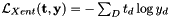• 2. Mse : per-frame mean-square-error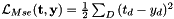• where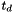is element of target vector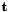,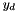is element of DNN-output vector, and D is the dimension of DNN-output
• nnet-forward.cc : forwards data through neural network, using CPU by default
• See options:
• –apply-log : produce log of NN-outputs (i.e. get log-posteriors)
• –no-softmax : removes soft-max from the model (decoding with pre-softmax values leads to the same lattices as with log-posteriors)
• –class-frame-counts : counts to calculate log-priors, which get subtracted from the acoustic scores (a typical trick in hybrid decoding).
• rbm-train-cd1-frmshuff.cc : train RBM using CD1, passes through training data several times while scheduling learning-rate / momentum internally
• nnet-train-mmi-sequential.cc : MMI / bMMI DNN training
• nnet-train-mpe-sequential.cc : MPE / sMBR DNN training

# Other tools

• nnet-copy.cc converts nnet to ASCII format by using –binary=false, can also be used to remove components

# Showing the network topology with nnet-info

The following print from nnet-info.cc shows the "feature_transform" corresponding to '–feat-type plain' of steps/nnet/train.sh, it contains 3 components:

• <Splice> which splices features to contain left/right context by using frames with offsets relative to the central frame [ -5 -4 -3 -2 -1 0 1 2 3 4 5 ]
• <Addshift> which shifts features to have zero mean
• <Rescale> which scales features to have unit variance
• Note: we read low-dimensional features from disk, expansion to high dimensional features is done on-the-fly by "feature_transform", this saves both hard-disk space and reading throughput
$nnet-info exp/dnn5b_pretrain-dbn_dnn/final.feature_transform num-components 3 input-dim 40 output-dim 440 number-of-parameters 0.00088 millions component 1 : <Splice>, input-dim 40, output-dim 440, frame_offsets [ -5 -4 -3 -2 -1 0 1 2 3 4 5 ] component 2 : <AddShift>, input-dim 440, output-dim 440, shift_data ( min -0.265986, max 0.387861, mean -0.00988686, variance 0.00884029, skewness 1.36947, kurtosis 7.2531 ) component 3 : <Rescale>, input-dim 440, output-dim 440, scale_data ( min 0.340899, max 1.04779, mean 0.838518, variance 0.0265105, skewness -1.07004, kurtosis 0.697634 ) LOG (nnet-info:main():nnet-info.cc:57) Printed info about exp/dnn5b_pretrain-dbn_dnn/final.feature_transform  The next print shows a neural network with 6 hidden layers: • each layer is composed of 2 components, typically the <AffineTransform> and a nonlinearity <Sigmoid> or <Softmax> • for each <AffineTransform> some statistics are shown (min, max, mean, variance, ...) separately for weights and bias $ nnet-info exp/dnn5b_pretrain-dbn_dnn/final.nnet
num-components 14
input-dim 440
output-dim 3370
number-of-parameters 28.7901 millions
component 1 : <AffineTransform>, input-dim 440, output-dim 2048,
linearity ( min -8.31865, max 12.6115, mean 6.19398e-05, variance 0.0480065, skewness 0.234115, kurtosis 56.5045 )
bias ( min -11.9908, max 3.94632, mean -5.23527, variance 1.52956, skewness 1.21429, kurtosis 7.1279 )
component 2 : <Sigmoid>, input-dim 2048, output-dim 2048,
component 3 : <AffineTransform>, input-dim 2048, output-dim 2048,
linearity ( min -2.85905, max 2.62576, mean -0.00995374, variance 0.0196688, skewness 0.145988, kurtosis 5.13826 )
bias ( min -18.4214, max 2.76041, mean -2.63403, variance 1.08654, skewness -1.94598, kurtosis 29.1847 )
component 4 : <Sigmoid>, input-dim 2048, output-dim 2048,
component 5 : <AffineTransform>, input-dim 2048, output-dim 2048,
linearity ( min -2.93331, max 3.39389, mean -0.00912637, variance 0.0164175, skewness 0.115911, kurtosis 5.72574 )
bias ( min -5.02961, max 2.63683, mean -3.36246, variance 0.861059, skewness 0.933722, kurtosis 2.02732 )
component 6 : <Sigmoid>, input-dim 2048, output-dim 2048,
component 7 : <AffineTransform>, input-dim 2048, output-dim 2048,
linearity ( min -2.18591, max 2.53624, mean -0.00286483, variance 0.0120785, skewness 0.514589, kurtosis 15.7519 )
bias ( min -10.0615, max 3.87953, mean -3.52258, variance 1.25346, skewness 0.878727, kurtosis 2.35523 )
component 8 : <Sigmoid>, input-dim 2048, output-dim 2048,
component 9 : <AffineTransform>, input-dim 2048, output-dim 2048,
linearity ( min -2.3888, max 2.7677, mean -0.00210424, variance 0.0101205, skewness 0.688473, kurtosis 23.6768 )
bias ( min -5.40521, max 1.78146, mean -3.83588, variance 0.869442, skewness 1.60263, kurtosis 3.52121 )
component 10 : <Sigmoid>, input-dim 2048, output-dim 2048,
component 11 : <AffineTransform>, input-dim 2048, output-dim 2048,
linearity ( min -2.9244, max 3.0957, mean -0.00475199, variance 0.0112682, skewness 0.372597, kurtosis 25.8144 )
bias ( min -6.00325, max 1.89201, mean -3.96037, variance 0.847698, skewness 1.79783, kurtosis 3.90105 )
component 12 : <Sigmoid>, input-dim 2048, output-dim 2048,
component 13 : <AffineTransform>, input-dim 2048, output-dim 3370,
linearity ( min -2.0501, max 5.96146, mean 0.000392621, variance 0.0260072, skewness 0.678868, kurtosis 5.67934 )
bias ( min -0.563231, max 6.73992, mean 0.000585582, variance 0.095558, skewness 9.46447, kurtosis 177.833 )
component 14 : <Softmax>, input-dim 3370, output-dim 3370,
LOG (nnet-info:main():nnet-info.cc:57) Printed info about exp/dnn5b_pretrain-dbn_dnn/final.nnet


## Frame-weighted training

call steps/nnet/train.sh with option :

--frame-weights <weights-rspecifier>


where <weights-rspecifier> is typically ark file with float vectors with per-frame weights,

• the weights are used to scale gradients computed on single frames, which is useful in confidence-weighted semi-supervised training,
• or weights can be used to mask-out frames we don't want to train with by generating vectors composed of weights 0, 1

## Training with external targets

call steps/nnet/train.sh with options

--labels <posterior-rspecifier> --num-tgt <dim-output>


while ali-dirs and lang-dir become dummy dirs. The "<posterior-rspecifier>" is typically ark file with Posterior stored, and the "<dim-output>" is the number of neural network outputs. Here the Posterior does not have probabilistic meaning, it is simply a data-type carrier for representing the targets, and the target values can be arbitrary float numbers.

When training with a single label per-frame (i.e. the 1-hot encoding), one can prepare an ark-file with integer vectors having the same length as the input features. The elements of this integer vector encode the indices of the target class, which corresponds to the target value being 1 at the neural network output with that index. The integer vectors get converted to Posterior using ali-to-post.cc, and the integer vector format is simple:

utt1 0 0 0 0 1 1 1 1 1 2 2 2 2 2 2 ... 9 9 9
utt2 0 0 0 0 0 3 3 3 3 3 3 2 2 2 2 ... 9 9 9


In the case of multiple non-zero targets, one can prepare the Posterior directly in ascii format

• each non-zero target value is encoded by a pair <int32,float>, where int32 is the index of NN output (starting by 0) and float is the target-value
• each frame (i.e. datapoint) is represented by values in brackets [ ... ], we see that the <int32,float> pairs get concatenated
utt1 [ 0 0.9991834 64 0.0008166544 ] [ 1 1 ] [ 0 1 ] [ 111 1 ] [ 0 1 ] [ 63 1 ] [ 0 1 ] [ 135 1 ] [ 0 1 ] [ 162 1 ] [ 0 1 ] [ 1 0.9937257 12 0.006274292 ] [ 0 1 ]


The external targets are used in the autoencoder example egs/timit/s5/local/nnet/run_autoencoder.sh

## Mean-Square-Error training

call steps/nnet/train.sh with the options

--train-tool "nnet-train-frmshuff --objective-function=mse"
--proto-opts "--no-softmax --activation-type=<Tanh> --hid-bias-mean=0.0 --hid-bias-range=1.0"


the mean-square error training is used in autoencoder example egs/timit/s5/local/nnet/run_autoencoder.sh

## Training with tanh

call steps/nnet/train.sh with option

--proto-opts "--activation-type=<Tanh> --hid-bias-mean=0.0 --hid-bias-range=1.0"


the optimal learning rate is smaller than with sigmoid, usually 0.00001 is good

## Conversion of a DNN model between nnet1 -> nnet2

In Kaldi, there are 2 DNN setups Karel's (this page) and Dan's Dan's DNN implementation. The setups use incompatible DNN formats, while there is a converter of Karel's DNN into Dan's format.

• The example script is : egs/rm/s5/local/run_dnn_convert_nnet2.sh, model conversion
• The model-conversion script is : steps/nnet2/convert_nnet1_to_nnet2.sh, it is calling the model-conversion binary : nnet1-to-raw-nnet.cc
• For list of supported components see ConvertComponent.

# The C++ code

The nnet1 code is located at src/nnet, the tools are at src/nnetbin. It is based on src/cudamatrix.

## Neural network representation

The neural network consists of building blocks called Component, which can be for example AffineTransform or a non-linearity Sigmoid, Softmax. A single DNN "layer" is typically composed of two components : the AffineTransform and a non-linearity.

The class which represents neural networks: Nnet is holding a vector of Component pointers Nnet::components_. The important methods of Nnet are :

• Nnet::Propagate : propagates input to output, while keeping per-component buffers that are needed for gradient computation
• Nnet::Backpropagate : back-propagates the loss derivatives, updates the weights
• Nnet::Feedforward : propagation, while using two flipping buffers to save memory
• Nnet::SetTrainOptions : sets the training hyper-parameters (i.e. learning rate, momentum, L1, L2-cost)

For debugging purposes, the components and buffers are accessible via Nnet::GetComponent, Nnet::PropagateBuffer, Nnet::BackpropagateBuffer.

## Extending the network by a new component

When creating a new Component, you need to use one of the two interfaces:

1. Component : a building block, contains no trainable parameters (see example of implementation nnet-activation.h)

2. UpdatableComponent : child of Component, a building block with trainable parameters (implemented for example in nnet-affine-transform.h)

The important virtual methods to implement are (not a complete list) :

Extending the NN framework by a new component is not too difficult, you need to :

1. define new entry to Component::ComponentType

2. define a new line in table Component::kMarkerMap